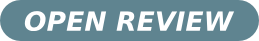# Expansions for eigenfunction and eigenvalues of large-$n$ Toeplitz matrices

## Authors

• Leo P. Kadanoff The James Franck Institute, The University of Chicago

## Keywords:

Mathematical Physics

## Abstract

This paper constructs methods for finding convergent expansions for eigenvectors and eigenvalues of large-$n$ Toeplitz matrices based on a situation in which the analogous infinite-$n$ matrix would be singular. It builds upon work done by Dai, Geary, and Kadanoff [H Dai et al., J. Stat. Mech. P05012 (2009)] on exact eigenfunctions for Toeplitz operators which are infinite-dimension Toeplitz matrices. One expansion for the finite-$n$ case is derived from the operator eigenvalue equations obtained by continuing the finite-$n$ Toeplitz matrix to plus infinity. A second expansion is obtained by continuing the finite-$n$ matrix to minus infinity. The two expansions work together to give an apparently convergent expansion for the finite-$n$ eigenvalues and eigenvectors, based upon a solvability condition for determining eigenvalues. The expansions involve an expansion parameter expressed as an inverse power of $n$. A variational principle is developed, which gives an approximate expression for determining eigenvalues. The lowest order asymptotics for eigenvalues and eigenvectors agree with the earlier work [H Dai et al., J. Stat. Mech. P05012 (2009)]. The eigenvalues have a $(\ln n)/n$ term as their leading finite-$n$ correction in the central region of the spectrum. The $1/n$ correction in this region is obtained here for the first time.

Received: 19 October 2009; Accepted: 29 September 2010; Edited by: A. G. Green; Reviewed by: T. Ehrhardt, Math. Dept., Univ. California, Santa Cruz, USA; DOI: 10.4279/PIP.0200032010-10-19

## How to Cite

Kadanoff, L. P. (2010). Expansions for eigenfunction and eigenvalues of large-$n$ Toeplitz matrices. Papers in Physics, 2, 020003. https://doi.org/10.4279/pip.020003

Articles